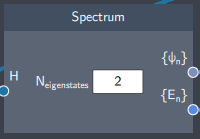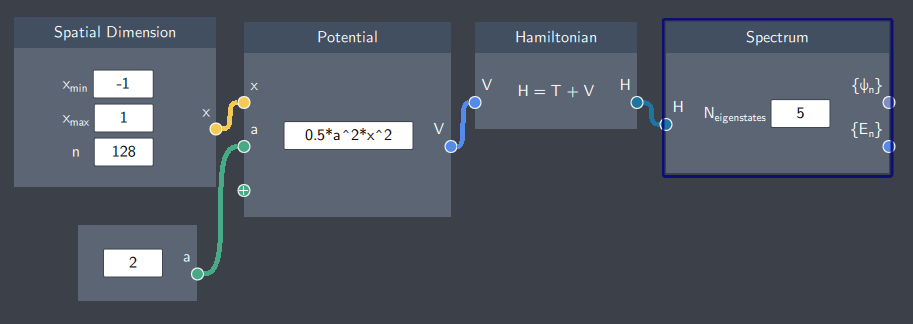# Spectrum

## Description

This node calculates the eigenstates and eigenvalues of the system defined by the Hamiltonian.## Input

The node has the following input:

• Hamiltonian ($H$): A Hamiltonian defined in a Hamiltonian node.

## Content

In the content field, $N_{eigenstates}$ corresponds to the number of eigenstates and eigenvalues that will be calculated and sent to the output. If this value is set to 1, the node calculates the first eigenstate and eigenvalue of the system, which is the ground state.

By default, this value is set at 2 and it can be changed to a higher number to output more than two eigenstates & eigenvalues.

## Output

• Spectrum of eigenstates ($\{ \psi_n \}$): The list of eigenstates of the system defined by the Hamiltonian.
• Spectrum of eigenvalues ($\{ E_n \}$): The list of eigenvalues of the corresponding eigenstates of the system.

## Example

In the example below, the spectrum of a harmonic oscillator is calculated. The content field reads ''5'' which means that the Spectrum node will output the first 5 eigenstates and eigenvalues of this system.